International
Tables for
Crystallography
Volume C
Mathematical, physical and chemical tables
Edited by E. Prince

International Tables for Crystallography (2006). Vol. C, ch. 8.4, pp. 702-703

## Section 8.4.1. The χ2 distribution

E. Princea and C. H. Spiegelmanb

aNIST Center for Neutron Research, National Institute of Standards and Technology, Gaithersburg, MD 20899, USA, and bDepartment of Statistics, Texas A&M University, College Station, TX 77843, USA

### 8.4.1. The χ2 distribution

| top | pdf |

We have seen [equation (8.1.2.1)] that the least-squares estimate is derived by finding the minimum value of a sum of terms of the form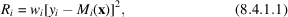and, further, that the precision of the estimate is optimized if the weight,, is the reciprocal of the variance of the population from which the observation is drawn,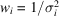. Using this relation, (8.4.1.1)can be written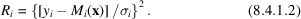Each term is the square of a difference between observed and calculated values, expressed as a fraction of the standard uncertainty of the observed value. But, by definition,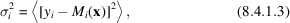where x has its unknown correct' value, so that <R> = 1, and the expected value of the sum of n such terms is n. It can be shown (Draper & Smith, 1981) that each parameter estimated reduces this expected sum by one, so that, for p estimated parameters,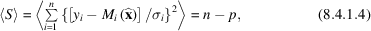whereis the least-squares estimate. The standard uncertainty of an observation of unit weight, also referred to as the goodness-of-fit parameter, is defined by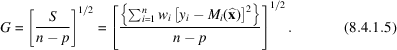From (8.4.1.4), it follows that <G> = 1 for a correct model with weights assigned in accordance with (8.4.1.2).

A value of G that is close to one, if the weights have been assigned by, is an indicator that the model is consistent with the data. It should be noted that it is not necessarily an indicator that the model is correct', because it does not rule out the existence of an alternative model that fits the data as well or better. An assessment of the adequacy of the fit of a given model depends, however, on what is meant by `close to one', which depends in turn on the spread of a probability density function for G. We saw in Chapter 8.1that least squares with this weighting scheme would give the best, linear, unbiased estimate of the model parameters, with no restrictions on the p.d.f.s of the populations from which the observations are drawn except for the implicit assumption that the variances of these p.d.f.s are finite. To construct a p.d.f. for G, however, it is necessary to make an assumption about the shapes of the p.d.f.s for the observations. The usual assumption is that these p.d.f.s can be described by the normal p.d.f.,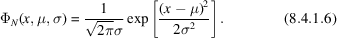The justification for this assumption comes from the central-limit theorem, which states that, under rather broad conditions, the p.d.f. of the arithmetic mean of n observations drawn from a population with mean μ and variance σ2 tends, for large n, to a normal distribution with mean μ and variance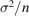. [For a discussion of the central limit theorem, see Cramér (1951).]

If we make the assumption of a normal distribution of errors and make the substitution z = (x − μ)/σ, (8.4.1.6)becomes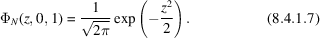The probability that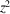will be less than χ2 is equal to the probability that z will lie in the interval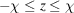, or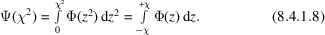Letting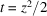and substituting in (8.4.1.7), this becomes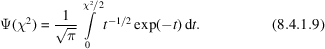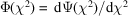, so that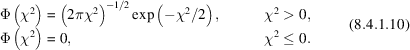The joint p.d.f. of the squares of two random variables,and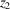, drawn independently from the same population with a normal p.d.f. is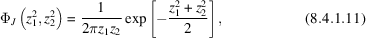and the p.d.f. of the sum,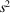, of these two terms is the integral over the joint p.d.f. of all pairs of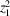and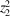that add up to.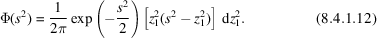This integral can be evaluated by use of the gamma and beta functions. The gamma function is defined for positive real x by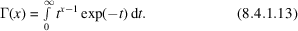Although this function is continuous for all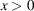, its value is of interest in the context of this analysis only for x equal to positive, integral multiples of 1/2. It can be shown that Γ(1/2) =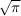, Γ(1) = 1, and Γ(x + 1) = xΓ(x). It follows that, for a positive integer, n, Γ(n) = (n −1)!, and that Γ(3/2) =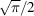, Γ(5/2) =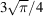, etc. The beta function is defined by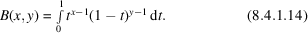It can be shown (Prince, 1994) that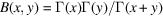. Making the substitution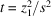, (8.4.1.12)becomes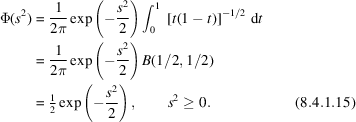By a similar procedure, it can be shown that, if χ2 is the sum of ν terms,,,,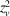, where all are drawn independently from a population with the p.d.f. given in (8.4.1.10), χ2 has the p.d.f.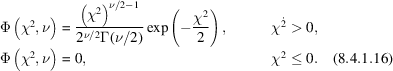The parameter ν is known as the number of degrees of freedom, but this use of that term must not be confused with the conventional use in physics and chemistry. The p.d.f. in (8.4.1.16)is the chi-squared distribution with ν degrees of freedom. Table 8.4.1.1gives the values of χ2/ν for which the cumulative distribution function (c.d.f.) Ψ(χ2, ν) has various values for various choices of ν. This table is provided to enable verification of computer codes that may be used to generate more extensive tables. It was generated using a program included in the statistical library DATAPAC (Filliben, unpublished). Fortran code for this program appears in Prince (1994).

 Table 8.4.1.1| top | pdf | Values of χ2/ν for which the c.d.f. ψ(χ2, ν) has the values given in the column headings, for various values of ν
ν0.5 0.9 0.95 0.99 0.995
1 0.4549 2.7055 3.8415 6.6349 7.8795
2 0.6931 2.3026 2.9957 4.6052 5.2983
3 0.7887 2.0838 2.6049 3.7816 4.2794
4 0.8392 1.9449 2.3719 3.3192 3.7151
6 0.8914 1.7741 2.0986 2.8020 3.0913
8 0.9180 1.6702 1.9384 2.5113 2.7444
10 0.9342 1.5987 1.8307 2.3209 2.5188
15 0.9559 1.4871 1.6664 2.0385 2.1868
20 0.9669 1.4206 1.5705 1.8783 1.9999
25 0.9735 1.3753 1.5061 1.7726 1.8771
30 0.9779 1.3419 1.4591 1.6964 1.7891
40 0.9834 1.2951 1.3940 1.5923 1.6692
50 0.9867 1.2633 1.3501 1.5231 1.5898
60 0.9889 1.2400 1.3180 1.4730 1.5325
80 0.9917 1.2072 1.2735 1.4041 1.4540
100 0.9933 1.1850 1.2434 1.3581 1.4017
120 0.9945 1.1686 1.2214 1.3246 1.3638
140 0.9952 1.1559 1.2044 1.2989 1.3346
160 0.9958 1.1457 1.1907 1.2783 1.3114
200 0.9967 1.1301 1.1700 1.2472 1.2763

The quantity (np)G is the sum of n terms that have mean value (np)/n. Because the process of determining the least-squares fit establishes p relations among them, however, only (np) of the terms are independent. The number of degrees of freedom is therefore ν = (np), and, if the model is correct, and the terms have been properly weighted, χ2 = (np)G2 has the chi-squared distribution with (np) degrees of freedom. In crystallography, the number of degrees of freedom tends to be large, and the p.d.f. for G correspondingly sharp, so that even rather small deviations from G2 = 1 should cause one or both of the hypotheses of a correct model and appropriate weights to be rejected. It is common practice to assume that the model is correct, and that the weights have correct relative values, that is that they have been assigned by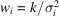, where k is a number different from, usually greater than, one. G is then taken to be an estimate of k, and all elements of (ATWA)−1 (Section 8.1.2) are multiplied by G2 to get an estimated variance–covariance matrix. The range of validity of this procedure is limited at best. It is discussed further in Chapter 8.5.

### References

Cramér, H. (1951). Mathematical methods of statistics. Princeton, NJ: Princeton University Press.
Draper, N. & Smith, H. (1981). Applied regression analysis. New York: John Wiley.
Prince, E. (1994). Mathematical techniques in crystallography and materials science, 2nd ed. Berlin/Heidelberg/New York/London/Paris/Tokyo/Hong Kong/Barcelona/Budapest: Springer-Verlag.